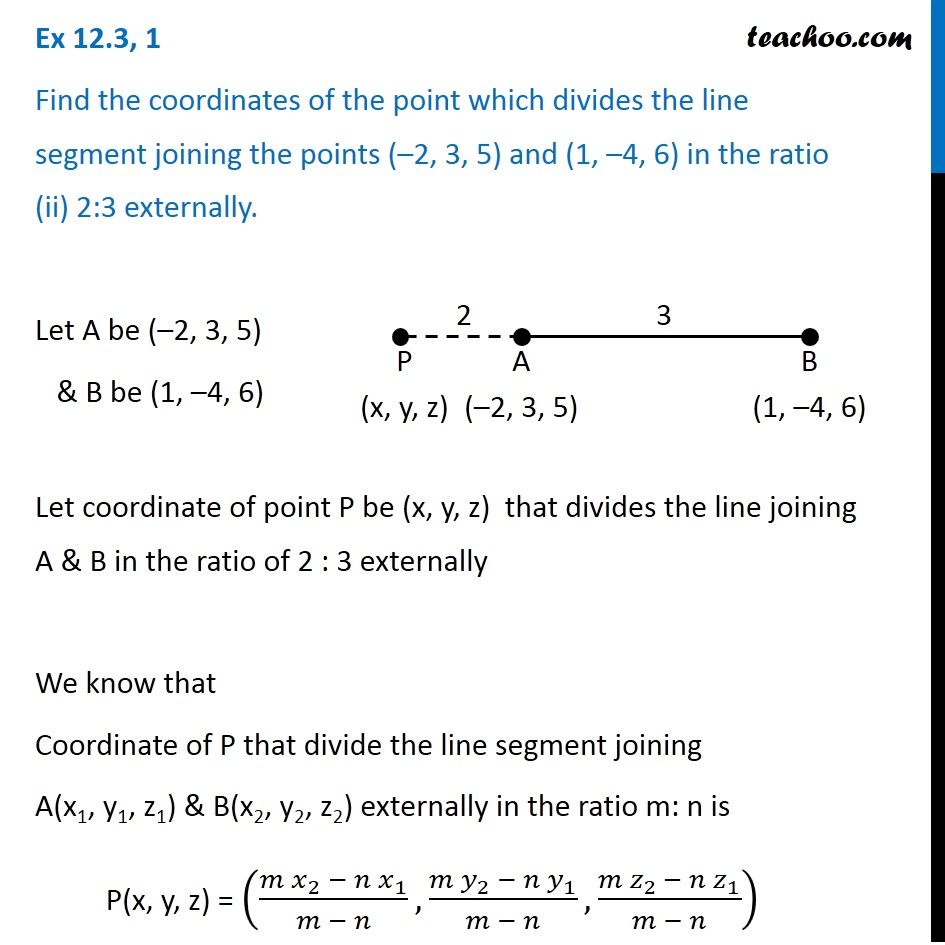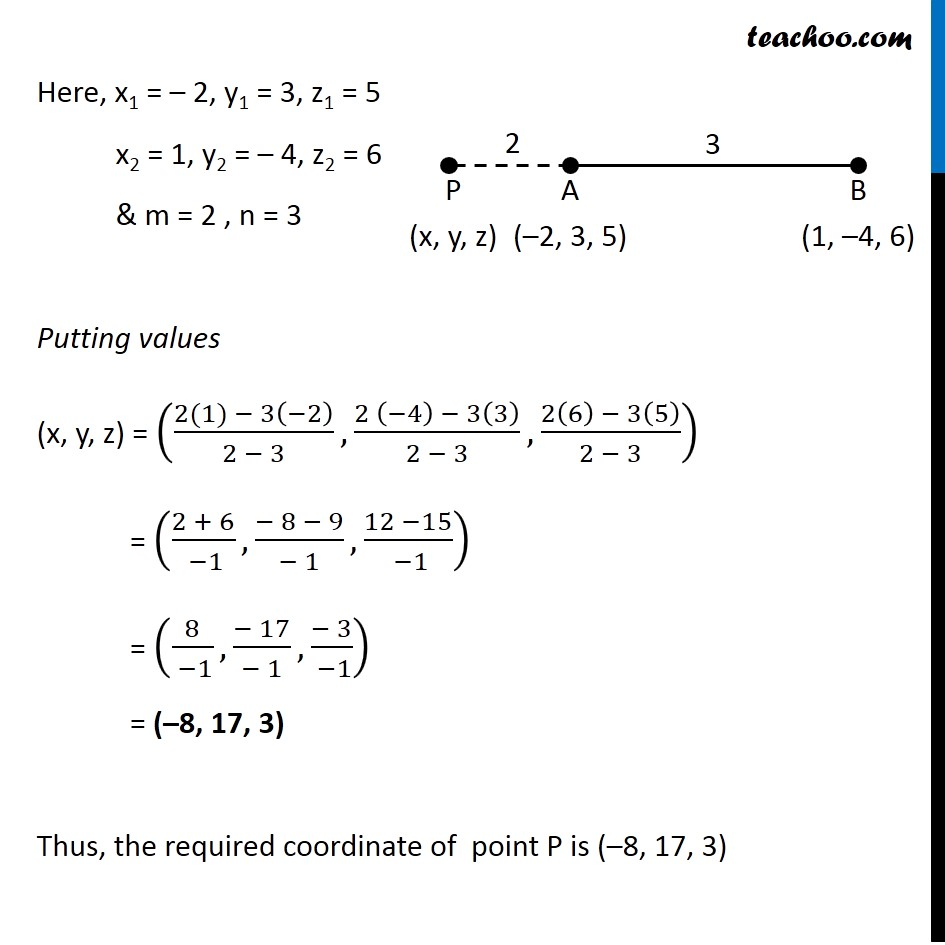Section Formula in 3D Geometry

Chapter 11 Class 11 - Intro to Three Dimensional Geometry
Serial order wiseLearn in your speed, with individual attention - Teachoo Maths 1-on-1 Class

### Transcript

Question 1 Find the coordinates of the point which divides the line segment joining the points (–2, 3, 5) and (1, –4, 6) in the ratio (ii) 2:3 externally. Let A be (–2, 3, 5) & B be (1, –4, 6) Let coordinate of point P be (x, y, z) that divides the line joining A & B in the ratio of 2 : 3 externally We know that Coordinate of P that divide the line segment joining A(x1, y1, z1) & B(x2, y2, z2) externally in the ratio m: n is P(x, y, z) = ((〖𝑚 𝑥〗_2 −〖 𝑛 𝑥〗_1)/(𝑚 − 𝑛),(〖𝑚 𝑦〗_2 −〖 𝑛 𝑦〗_1)/(𝑚 − 𝑛),(〖𝑚 𝑧〗_(2 )−〖 𝑛 𝑧〗_1)/(𝑚 − 𝑛)) Here, x1 = – 2, y1 = 3, z1 = 5 x2 = 1, y2 = – 4, z2 = 6 & m = 2 , n = 3 Putting values (x, y, z) = ((2(1) − 3(−2))/(2 − 3),(2 (−4) − 3(3))/(2 − 3),(2(6) − 3(5))/(2 − 3)) = ((2 + 6)/( −1),(− 8 − 9)/(− 1),(12 −15)/( −1)) = (8/( −1),(− 17)/(− 1),(− 3)/( −1)) = (–8, 17, 3) Thus, the required coordinate of point P is (–8, 17, 3)